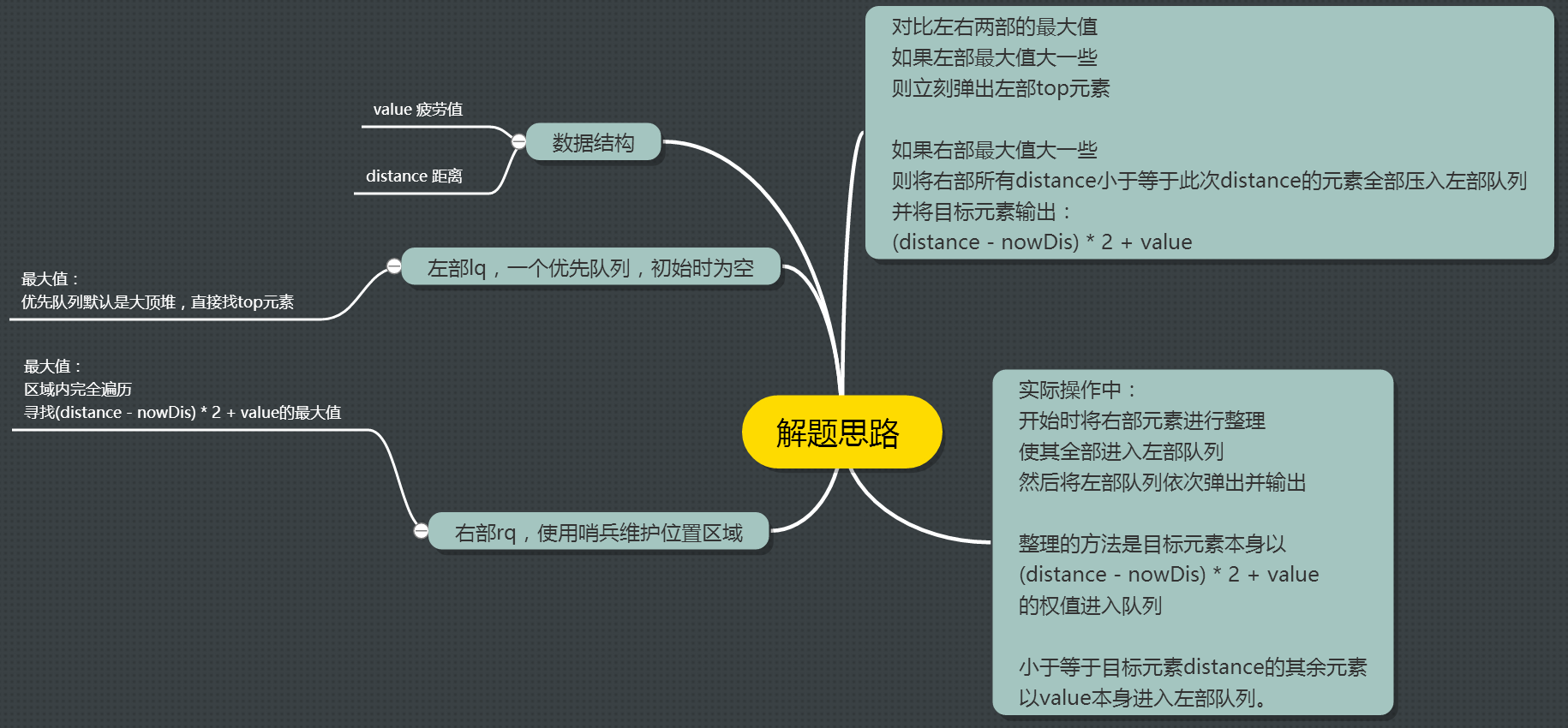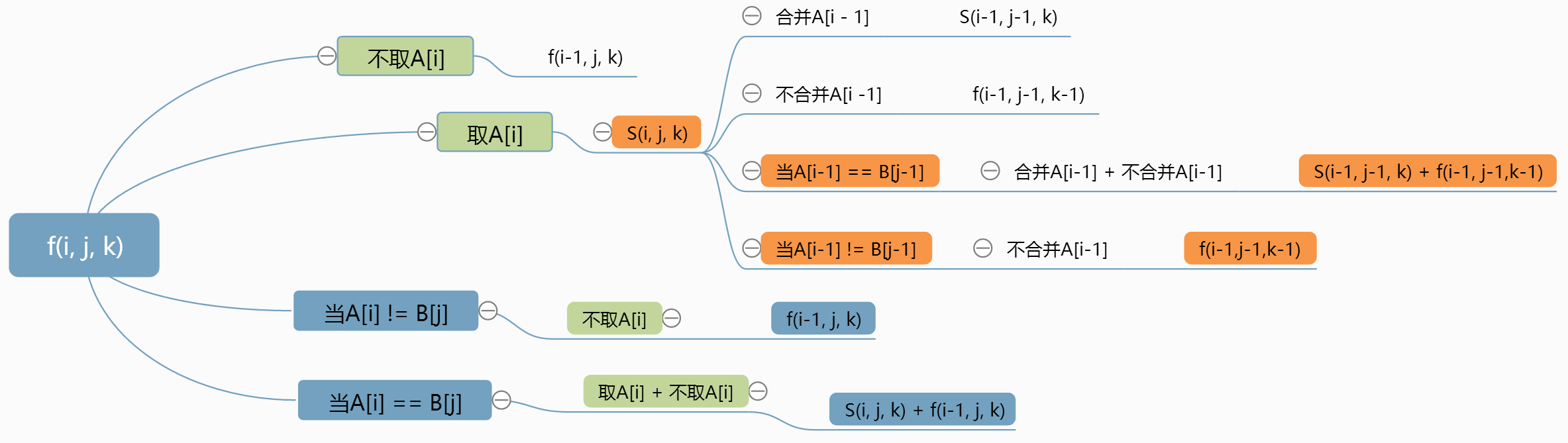## NOIP2015 推销员 解题报告

C++中pair的用法

#### 解题思路：

##### 每次对左部和右部的最大值进行对比：
• 如果左部最大值大一些，则此时选择左部元素（推销员已走过的房间），将左部元素的value值输出，并弹出这个元素。
• 如果右部最大值大一些，则此时选择右部元素（推销员还没到过的坐标），将右部元素的`(distance - nowDis) * 2 + value`值输出，同时记录目标元素的distance值，并将右部元素中所有小于等于此distance值的节点全部压入左部队列，压入权值为每个元素的value值。
##### 寻找最大值的方法：
• 左部元素寻找最大值的方法：直接使用优先队列的特性，lq的top元素。

• 右部元素寻找最大值的方法：在rq中依次遍历，寻找最大的`(distance - nowDis) * 2 + value`##### 代码：
``````/**
* NOIP2015 推销员
* 学习使用pair泛型进行组合，而不需要自己写struct
* 学习使用priority_queue优先队列（默认为最大堆）
*/
#include <stdio.h>
#include <vector>
#include <queue>

using namespace std;

int main()
{
int N;
scanf("%d", &N);
pair<int, int> nodes[N];
for(int i = 0; i < N; i++){
scanf("%d", &nodes[i].second);      //读入dis数据
}
for(int i = 0; i < N; i++){
scanf("%d", &nodes[i].first);       //读入value数据
}

priority_queue< pair<int, int> > lq;    //左部优先队列

int nowPos = 0, nowDis = 0, nowResult = 0, tempPos, tempMXPos, tempResult, tempMXResult;

//将nowPos不断向N逼近，迫使右部数据全部进入左部队列，最后整体输出左部队列
while(nowPos < N){
//找到本次登记的最大result和最大dis
tempMXResult = 0;
for(tempPos = nowPos; tempPos < N; tempPos++){
tempResult = (nodes[tempPos].second - nowDis) * 2 + nodes[tempPos].first;
if( tempResult >= tempMXResult ){
tempMXResult = tempResult;
tempMXPos = tempPos;
}
}
nowDis = nodes[tempMXPos].second;
//tempMXPos的权值为tempMXResult，其余小于nowDis的右部元素权值为first，将这些元素全部压入优先队列lq中
for(tempPos = nowPos; nodes[tempPos].second <= nowDis && tempPos < N; tempPos++){
if(tempPos == tempMXPos){
nodes[tempPos].first = tempMXResult;
}
lq.push(nodes[tempPos]);
}
nowPos = tempPos;
}

while(!lq.empty()){
nowResult += lq.top().first;
printf("%d\n", nowResult);
lq.pop();
}

return 0;
}
``````

## NOIP2015子串问题解题报告

#### 样例分析

``````6 3 1
aabaab
aab
6 3 2
aabaab
aab
6 3 3
aabaab
aab
``````

aab aab
aab aab
(两种方案)

a ab aab
a aba ab
a a ba ab
aab a ab
aa b aab
aa baa b
aab aa b
(七种方案)

a a b aab
a a baa b
a ab a a b
a aba a b
a a b a a b
a a ba a b
aab a a b
(七种方案)

#### 推导f方程

PS：k=0时，无视其他所有值，f直接等于0，因为无法将主串取出0个子集出来构成子串。
f(5,2,2) = ?

``````if(A[i] == B[j]){    //取A[i]和不取A[i]
f(i,j,k) = s(i,j,k) + f(i-1, j, k);
}else{                //不取A[i]
f(i,j,k) = f(i-1, j, k);
}
``````

#### 推导s方程

s的定义是取A[i]这个字符时，从A[0,i]中取k个子集构成B[0-j]的方法数，也就是潜在的现状就是A[i] == B[j]

No,No,No,取不取A这个问题问得不好，会产生二义性，我们需要问一个更明确的问题：合并A[4,5]使其作为一个整体，还是不合并？

``````if(A[i-1] == B[j-1]){    //合并+不合并
s(i,j,k) = s(i-1, j-1, k) + f(i-1, j-1, k-1);
}else{                    //不合并
s(i,j,k) = f(i-1, j-1, k-1);
}
``````#### f,s都有了，下面用递归函数AC这道题吧

``````    //本来想贴代码的，想想只能A40%的代码也没人愿意看啊，还是算了。。。
``````

#### 滚动数组

``````if(A[i-1] == B[j-1]){    //合并+不合并
s(i,j,k) = s(i-1, j-1, k) + f(i-1, j-1, k-1);
}else{                    //不合并
s(i,j,k) = f(i-1, j-1, k-1);
}
``````

``````if(A[i] == B[j]){    //取A[i]和不取A[i] f(i,j,k) = s(i,j,k) + f(i-1, j, k);
if(A[i-1] == B[j-1]){    //合并+不合并
f(i,j,k) = s(i-1, j-1, k) + f(i-1, j-1, k-1) + f(i-1, j, k);
}else{            //不合并
f(i,j,k) = f(i-1, j-1, k-1) + f(i-1, j, k);
}
}else{                //不取A[i]
f(i,j,k) = f(i-1, j, k);
}
``````

f[N][M][K]同样看做一个立方体，那么它在层层往上搭建的过程中，不光用到了k层的二维平面，还用到了k-1层的二维平面，好说，只要他不是在立方体里到处乱跑就行，不就是用到下一层吗，那我们同样没必要开辟f[N][M][K],我们只需要开辟f[N][M]就行了，然后用一个flag从0到1，从1再到0，轮流交替使用f[i][j][flag]就OK了，滚动数组的技巧完美get。

#### 最终编码

``````#include <stdio.h>
#include <stdlib.h>
#include <stdbool.h>

char *A, *B;

int main()
{
int n,m,K;
scanf("%d %d %d", &n, &m, &K);
int f[n][m];
int s[n][m];
A = (char*)malloc( (n+1) * sizeof(n));
B = (char*)malloc( (m+1) * sizeof(m));
scanf("%s", A);
scanf("%s", B);

/*
* ACM比赛中，1s对应的计算量大约是4*10^8
* 因此O(n*m*K) = 1000 * 200 * 1000是可以接受的
*/
int k,i,j;
bool flag = false;
for(k = 1; k<=K; k++){
for(i = 0; i<n; i++)
for(j = k-1; j<=i && j<m; j++){

if(i < j || j < (k-1)){
f[i][j][flag?1:0] = 0;
s[i][j] = 0;
continue;
}

if(j == 0 && k ==1){
s[i][j] = A[i] == B[j] ? 1 : 0;
f[i][j][flag?1:0] = (long long)((long long)s[i][j] + ((k <= 0 || i<1 || j<0 || i<j || j<(k-1))?0:(long long)f[i-1][j][flag?1:0])) % 1000000007;
continue;
}

if(A[i] == B[j]){
if(i>0 && j>0){
if(A[i-1] == B[j-1])
s[i][j] = (long long)((j<k?0:s[i-1][j-1]) + ((k<=1)?0:f[i-1][j-1][flag?0:1])) % 1000000007;
else
s[i][j] = (k<=1)?0:f[i-1][j - 1][flag?0:1];
}
f[i][j][flag?1:0] = (long long)(s[i][j] + ((k <= 0 || i<1 || j<0 || i<=j || j<(k-1))?0:f[i-1][j][flag?1:0]) ) % 1000000007;
}else{
f[i][j][flag?1:0] = i==0?0:f[i - 1][j][flag?1:0];
}
}
flag = !flag;
}

printf("%d\n", f[n - 1][m - 1][flag?0:1]);

return 0;
}

/**************************************************************
Problem: 2241
User: newflydd
Language: C
Result: 正确
Time:396 ms
Memory:3168 kb
****************************************************************/
``````

• 现居住地 江苏 ● 泰州 ● 姜堰
• 创建了 Jblog 开源博客系统
• 坚持十余年的 独立博客 作者
• 大学本科毕业后就职于 中国电信江苏泰州分公司，前两年从事Oracle数据库DBA工作，两年后公司精简技术人员，被安排到农村担任支局长（其本质是搞销售），于2016年因志向不合从国企辞职，在小城镇找了一份程序员的工作。
• 在 Git OSChina 上积极参与开源社区
•